Three-phase Induction Motor: Squirrel cage induction motor, Slip ring (or phase wound) induction motor, stationary stator, revolving rotor, Squirrel-cage, slip ring and Basic Working Principle of an Induction Motor ~ MECHTECH GURU

# Three-phase Induction Motor

Induction Motors are the most commonly used motors in most of the applications. These are also called as Asynchronous Motors, because an induction motor always runs at a speed lower than synchronous speed. The induction motor is made up of the stator, or stationary windings, and the rotor. The stator consists of a series of wire windings of very low resistance permanently attached to the motor frame. When a voltage is applied to the stator winding terminals, a magnetic field is developed in the windings. By the way the stator windings are arranged, the magnetic field appears to synchronously rotate electrically around the inside of the motor housing.

Depending upon the input supply there are basically two types of induction motor,

## (A) Single phase induction motor

Split phase induction motor

Capacitor start induction motor

Capacitor start capacitor run induction motor

## (B) Three phase induction motor

Squirrel cage induction motor

Slip ring (or phase wound) induction motor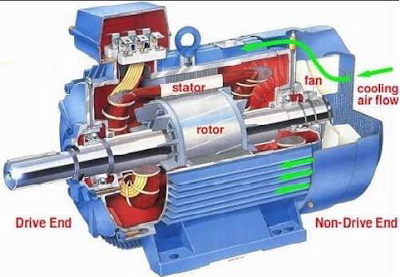Three phase induction motor

Construction

An induction motor has two main parts:

## a) a stationary stator

It consisting of a steel frame that supports a hollow, cylindrical core, constructed from stacked laminations having a number of evenly spaced slots, providing the space for the stator winding.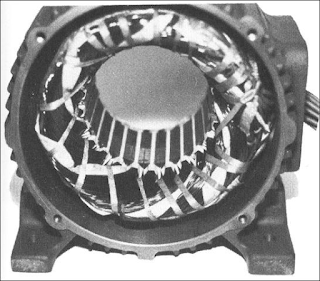Stator

## b) a revolving rotor

It composed of punched laminations, stacked to create a series of rotor slots, providing space for the rotor winding. There are two types of rotors, squirrel cage type and slip ring type.

Squirrel-cage: conducting bars laid into slots and shorted at both ends by shorting rings.

Slip ring: complete set of three-phase windings exactly as the stator. Usually Y-connected, the ends of the three rotor wires are connected to 3 slip rings on the rotor shaft. In this way, the rotor circuit is accessible.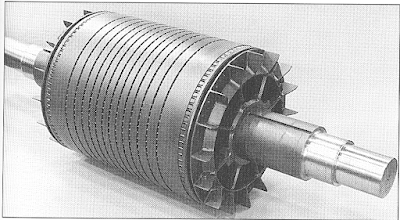Squirrel cage rotor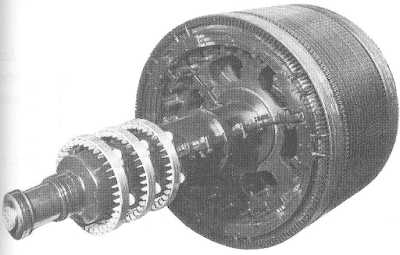Slip ring rotor

## Basic Working Principle of an Induction Motor

As a general rule, conversion of electric power into mechanical power takes place in the rotating part of an electric motor. In a DC motor, the electric power is conducted directly to the armature through brushes and commutator. Hence, in this sense a dc motor can be called a conduction motor. However, in ac motors, the rotor does not receive electric power by conduction but by induction in exactly the same way as the secondary of a two winding transformer receives its power from the primary. That is why such motors are known as induction motors.

When the three phase stator windings are fed by a three phase supply then, a magnetic flux of constant magnitude, but rotating at synchronous speed, is set up. The flux passes through the air gap, sweeps past the rotor surface and so cuts the rotor conductors which, as yet, are stationary. Due to the relative speed between the rotating flux and stationary conductors, an emf is induced in the later, according to Faraday’s laws of electro-magnetic induction. The frequency of the induced emf is same as the supply frequency. Its magnitude is proportional to the relative velocity between the flux and the conductors and its direction is given by Fleming’s Right-hand rule. Since the rotor bars or conductors form a closed circuit, rotor current is produced whose direction, as given by Lenz’s law, is such as to oppose the very cause producing it. In this case, the cause which produces the rotor current is the relative velocity between the rotating flux of the stator and the stationary rotor conductors. Hence, to reduce the relative speed, the rotor starts running in the same direction as that of the flux and tries to catch up with the rotating flux.

## Slip

In practice, the motor never succeeds in catching up with the stator fiel. If it really did so, then there would be no relative speed between the two, hence no rotor emf, no rotor current and so there will be no torque to maintain rotation. That is why the rotor runs at a speed which is always less than the speed of the stator field. The difference in speed depends upon the load on the motor.

The difference between the synchronous speed and the actual speed of the rotor is known as slip. It is expressed as a percentage of the synchronous speed.

It may be noted here that revolving flux is rotating synchronously, relative to the stator (i.e. stationary space) but at slip speed relative to the motor.

## Frequency of Rotor Current

When the rotor is stationary, the frequency of rotor current is same as the supply frequency. But when the rotor starts revolving, then the frequency depends upon the relative speed or on slip speed.  Let at any slip-speed, the frequency of the rotor current be f  . Then,

Starting Torque

The torque developed by the motor at the instant of starting is called starting torque.

Hence, torque under running condition is maximum when at that the slip which makes rotor

reactance per phase equal to rotor resistance per phase.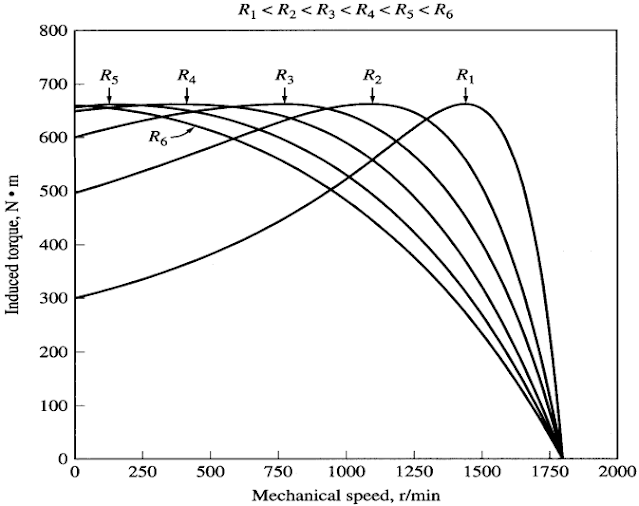Effect of rotor resistance on torque-speed characteristic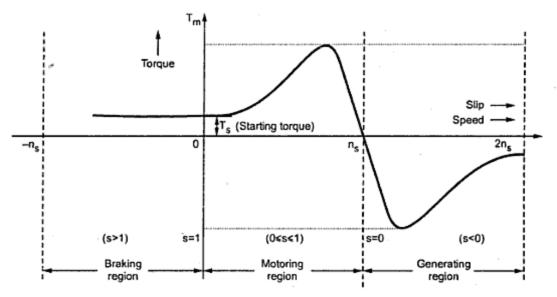Complete torque-speed characteristic of a three phase induction machine

Previous
Next Post »